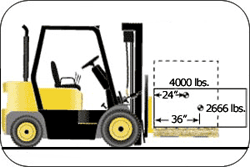Figure 1. As the center of gravity for the load moves forward, the lifting capacity for the forklift decreases.

The stated capacity of a forklift only applies to the load center indicated on the data plate. If the load is not centered at the specified position, the forklift's capacity will be reduced. Loads come in all shapes and sizes, not just symmetrical boxes. The load size, position, and weight distribution critically affect the forklift's capacity and the stability of the truck. Consider the following factors before engaging a load: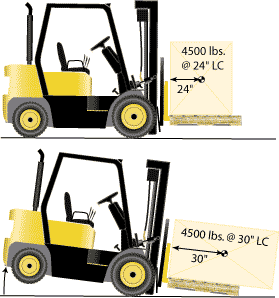Load weight, weight distribution, size, shape, and position are key factors affecting the stability of the forklift. Forklifts are designed to carry a capacity load at a standard load center, commonly 24 inches. This means that the forklift’s capacity was determined as if the load were a cube whose weight is evenly distributed (i.e., whose center of gravity is exactly in the center of the cube) and which is resting on a standard pallet having dimensions of 48 inches by 48 inches. With such a load, the horizontal distance from the center of the load to the vertical part of the forks would be 24 inches. Of course, most loads are not perfectly shaped cubes having their center of gravity exactly in the middle of the cube. To the extent that the load differs from this theoretical load — such as if it is irregularly shaped, has unbalanced weight distribution, or is not centered on the forks — the capacity may be reduced.

Potential Hazards

While arranging a load, be aware of the following:

• Tipover

• Loss of steering control (Shifting too much weight forward raises the rear wheels.)

• Collision

Requirements and Recommended Practices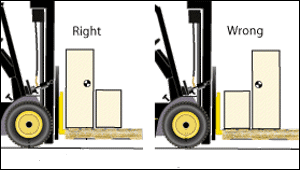• Do not exceed the capacity of the forklift that appears on the forklift's data plate (sometimes called the "nameplate"). If the load is oversized, irregularly shaped, or loaded incorrectly, the actual load center distance could exceed the stated load center distance, causing the truck's capacity to be exceeded. (Figure 2).

• Always minimize the distance from the front wheels to the load center. Load a large rectangular box widthwise across the forks of the truck as in Figure 2. Placing a large rectangular load lengthwise causes the load center to shift forward further away from the front wheels, exceeding the truck's capacity and lifting the rear wheels off the ground.

• Load as close to the front wheels as possible to minimize the load center distance. Load the heaviest part toward the mast. (Figure 3)

• Position the load in a way that will shorten the load center distance.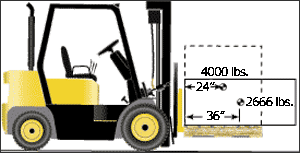Requirements and Recommended Practices

• If the stated load center is exceeded, compensate by reducing the weight of the load.

• Consult the forklift manufacturer’s instructions when handling large or unusually configured loads.

• Tip: Use field calculations to estimate the reduced lifting capacity if manufacturer’s instructions are not available. This calculation method will not produce exact load reduction figures. Use this method only as a guideline. The forklift manufacturer is the source of more precise information.

Field Calculation of Safe Load Capacity

Assume a situation where a forklift truck that has a 5,000 pound capacity at a 24 inch load center needs to handle a load whose center is 28 inches from the front face of the forks in the horizontal direction. The first thing to recognize is that the actual load center distance of 28 inches exceeds the standard load center distance of 24 inches on which the 5000 pound capacity is based, so the safe load capacity is actually less than 5000 pounds.

To estimate the truck's safe load capacity at a 28-inch load center, take the rated load center and divide it by the actual load center. Then multiply this number by the stated capacity to get the new approximate safe load capacity:

24 in/28 in x 5,000 lb = 4,285 lb (approximate safe load capacity)

Using the example in Figure 4, take the stated standard load center of 24 inches and divide it by the actual load center of 36 inches. Multiply this number by the stated capacity of 4,000 lb to get the new approximate safe load capacity:

24 in/36 in x 4,000 lb = 2,666 (approximate safe load capacity)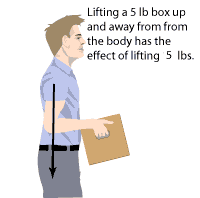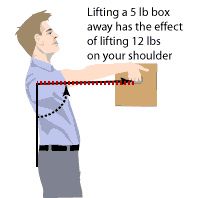The way in which weight is distributed changes the amount of weight the lift truck will safely carry. You can experience this for yourself by doing the following activity:

Lift a 5 pound box. As you extend your arms, the center of the box’s weight moves a greater distance from your body, so the box feels heavier and you will tend to fall forward. The same idea of increasing the load center distance applies to a playground see-saw: the farther you sit from the middle, the more you increase the load center distance and the more force you put on that end. The same principle—increasing the load center distance—can cause a forklift to tipover.

When the load center distance increases, it is actually increasing something called the "Load Moment":

Load Moment is the product of the object's weight multiplied by the object’s distance from the fulcrum, which is a fixed point that acts as the pivot point. On a sit-down counterbalanced forklift, the fulcrum or pivot point is the axle of the front wheels. It is this product, or Load Moment, which determines how much overturning force is being applied to the forklift.

Load Moment = Weight X Distance

If a load is 60 inches long (30-inch load center) then the maximum that this load can weigh is:

72,000 inch-pounds / 30 in-load center = 2,400 pounds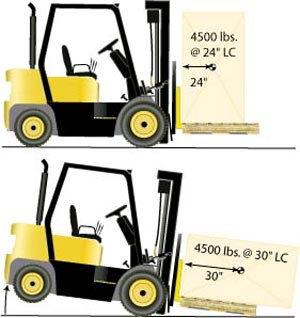Potential Hazards

While carrying a load near the maximum allowable capacity, be aware of the following:

• Danger of tipover

• Danger of being struck by falling load

Requirements and Recommended Practices

• Calculate a maximum allowable load moment to determine whether an unusual load, such as one that is longer than 48 inches (i.e., the load center distance would be greater than 24 inches) or that has an offset center of gravity (i.e., uneven weight distribution) can be handled safely.

• Minimize the load center distance measured from the back of the forks to the center of the load. This allows the forklift to carry more weight.

As illustrated in Figure 7, a truck that has a 4,500 pound capacity at a 24-inch load center will tipover if a 60-inch load is positioned lengthwise. Positioning the load in this way increases the load center distance to 30 inches and increases the load moment by 27,000 inch-pounds.

In Figure 7 the forklift safely carries the 4,500 pound load at a load center distance of 24 inches, but tips over when the load center increases to 30 inches. Here's the calculation: 30 inches X 4,500 pounds = 135,000 inch-pounds 24 inches X 4,500 pounds = 108,000 inch-pounds The load moment is increased by 27,000 inch-pounds.

If the load center distance is 30 inches, the only way to keep the maximum allowable load moment within 108,000 inch-pounds is to limit the weight of the load to 3600 pounds:

30 inches X 3600 pounds = 108,000 inch-pounds

• Use extra caution when handling extra heavy loads that may approach the truck's maximum capacity. For example, when handling a maximum load, the load should be carried at the lowest position possible, the truck should be accelerated slowly and evenly, and the forks should be tilted forward cautiously. However, there is no one rule for all situations.

• Maintain control of the vehicle at all times. The operator is responsible for handling the truck. Drive slower when carrying a load near the maximum allowable.

• Do not exceed the stated capacity of your truck. Know its mechanical limits.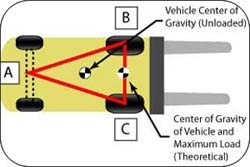Understanding why forklifts tipover and why loads become unstable and fall is very important to preventing accidents. Important factors that affect a forklift's balance are:

• Center of Gravity

• Shifting Center of Gravity

Center of Gravity

Potential Hazards

While operating a forklift, be aware of the following:

• Tipover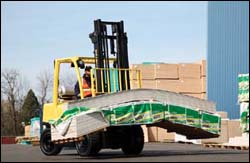Requirements and Recommended Practices

• Distribute the weight evenly when carrying irregular sized loads (Figure 9). [29 CFR 1910.178 App A]

• Keep the center of gravity of the load as near as possible to the center going horizontally across the forks.

• Keep the center of gravity of the load as near to the front wheels as possible.

Shifting Center of Gravity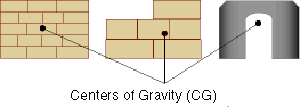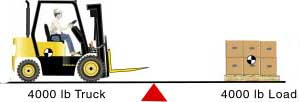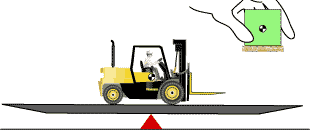All objects have a specific center of gravity. Gravity is a force that always pulls objects toward the earth's core. Center of gravity means the point on an object at which all of the object’s weight is concentrated and all of the parts balance each other. For symmetrical loads, the center of gravity is at the middle of the load in terms of the load’s length, width and height. (Figure 10). Since the capacity of the forklift is based on the assumption of a cube having the center of gravity in the middle, the shape and position of the actual load are key factors when determining whether a load can be carried safely.

Potential Hazards

Be aware of tipover or falling loads while:

• Operating a forklift, as the center of gravity shifts.

• Engaging or depositing a load.

Requirements and Recommended Practices:

• Handle loads within the capacity of the truck as stated on the data plate. [29 CFR 1910.178(o)(2)]

• Do not operate a forklift if the back wheels begin to lift off the ground. This is an indication that the forklift is overloaded. The center of gravity has shifted too far forward over the axle of the front wheels and the forklift may teeter on the wheels.

• Handle only stable or safely arranged loads. Exercise caution when handling off-center loads that cannot be centered. [29 CFR 1910.178(o)(1)]

• Tilt the mast forward cautiously when positioning the load onto the stack. [29 CFR 1910.178 App A]

• Never travel with the load elevated. Elevating the load increases the load center distance by shifting the center of gravity upward and forward, making the forklift and the load less stable (Figure 5).

• Adjust long or high (including multiple-tiered) loads which may affect capacity. [29 CFR 1910.178(o)(3)]

• Keep the center of gravity of the load as low to the ground and as close to the front wheels as possible:

• Carry the load at the lowest position possible, 4 to 6 inches from the ground.

• Tilt the mast back and position the heaviest part of the load against the carriage.

• Travel with the mast tilted back to stabilize the load.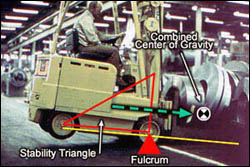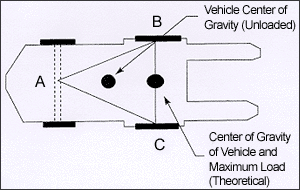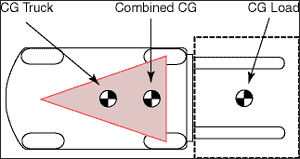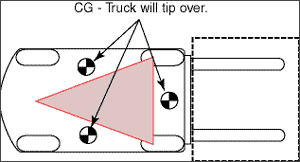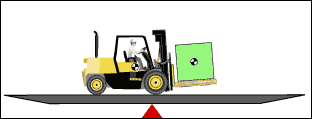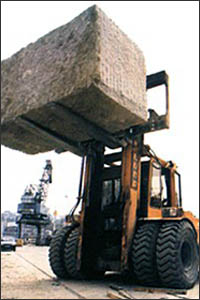Maintaining stability of a powered industrial truck is easier once you understand a few basic principles. There are many aspects of a vehicle's stability:

• The Stability Triangle

• Longitudinal Stability

• Lateral Stability

• Dynamic Stability

NOTE: This discussion focuses on sit-down counterbalanced forklifts. "Counterbalanced" means that the truck is weighted in the back with counterweight so that it will not tipover when the load is placed on the front. The counterweight is located between or behind the rear wheels and provides a weight in the back to "counterbalance" the weight of the load in the front. For a brief summary of other types of forklifts, see Types and Fundamentals.

The Stability Triangle

Almost all counterbalanced powered industrial trucks have a three-point suspension system, that is, the vehicle is supported at three points. This is true even if the vehicle has four wheels. The truck's steer axle is attached to the truck by a pivot pin in the axle's center. When this point is connected to the front wheels with imaginary lines, this three-point support forms a triangle called the stability triangle (Triangle ABC where Point A is the pivot point in the rear axle and Points B and C are the front wheels). (Figure 14) [A-4.1, 29 CFR 1910.178 Appendix A] So long as the center of gravity remains within this stability triangle, the truck is stable and will not tip over.

When the forklift is not loaded, the location of the forklift's center of gravity is the only factor to be considered in determining its stability. In Figure 14, the center of gravity is between the axle of the steer wheels at A and the drive wheels at B-C and it is marked with the arrow as the Vehicle Center of Gravity (Unloaded). In Figure 15, the combined center of gravity of the forklift and its maximum load shifts forward toward the load so that it is now located on the line representing the front axle, at the very edge of the stability triangle. While the loaded forklift is still theoretically stable, in practice the combined center of gravity should never reach this line because sudden stops, starts, and turns could shift the center of gravity further out and destabilize the forklift.

The Powered Industrial Truck Standard has a non-mandatory Appendix which more fully describes the forces involved and includes figures and definitions: [29 CFR 1910.178 Appendix A]

• Lateral stability is a truck's resistance to overturning sideways.

• Dynamic stability refers to the idea that an unloaded forklift's center of gravity and a loaded forklift's combined center of gravity can shift outside of the stability triangle as a result of certain movements, such as sudden stops and starts, turns, or operating on grades.

• Line of action is an imaginary vertical line through an object's center of gravity.

• Load center is the horizontal distance from the fork's or other attachment's vertical face to the line of action through the load's center of gravity.

• Moment is the product of the object's weight times the distance from a fixed point (usually the fulcrum). In the case of a powered industrial truck, the distance is measured from the point at which the truck will tipover to the object's line of action. The distance is always measured perpendicular to the line of action. [29 CFR 1910.178 Appendix A]

Requirements and Recommended Practices

• Maintain stability. Keep the combined center of gravity within the stability triangle.

• Do not accelerate rapidly or brake suddenly. Sudden changes in direction may also shift the combined center of gravity outside the vehicle's stability triangle and destabilize it.

• Do not turn rapidly. The combined center of gravity may shift outside the stability triangle and may cause the vehicle to tipover to the left or right.

• Never turn on a grade or ramp. Even a 10 percent grade may shift the combined center of gravity outside the stability triangle and cause the vehicle to roll over laterally.

• Cross an obstacle (railroad tracks, beam, pot hole) at a 45 degree angle, so both wheels do not elevate simultaneously.

• Maintain control of your vehicle at all times. Adjust your speed to match the conditions. Be aware and anticipate dangerous motions and avoid them.

• Consider the dynamic forces that result when the vehicle and load are put into motion. The weight's transfer and the resultant shift in the center of gravity due to the dynamic forces created when the machine is moving, braking, cornering, lifting, tilting, and lowering loads, etc., are important stability considerations. [A-7.1. 29 CFR 1910.178 Appendix A]

• When determining whether a load can be safely handled, the operator should exercise extra caution when handling loads that are close to the truck's stated capacity.

If an operator must handle a maximum load:
• The load should be carried at the lowest position possible,
• The truck should be accelerated slowly and evenly, and
• The forks should be tilted forward cautiously.

• However, no one rule can cover all eventualities. [A-7.2. 29 CFR 1910.178 Appendix A]